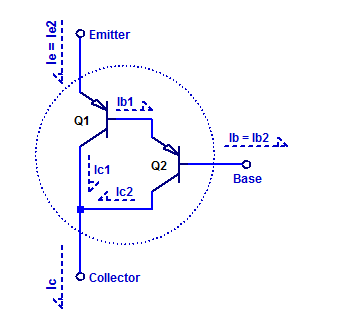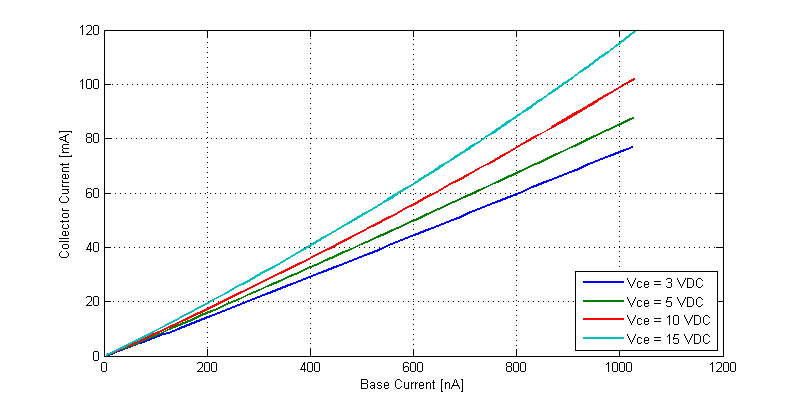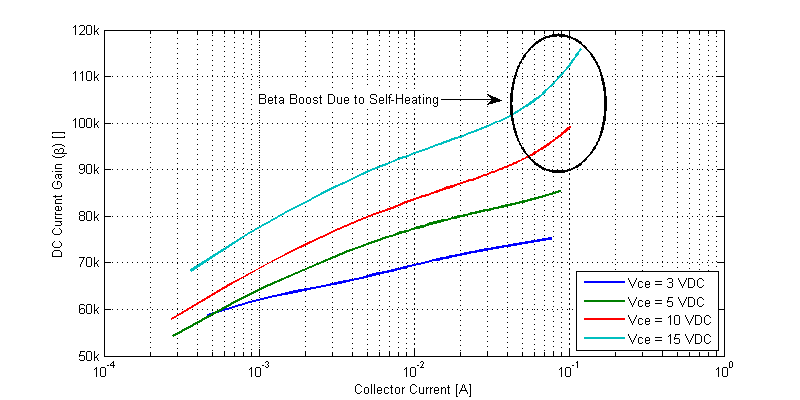# Darlington Current Gain

The darlington transistor arrangement consists of 2 cascaded bipolar transistors, in such a manor that the forward current gain of the first transistor is multiplied by the second.  A schematic of a PNP darlington transistor arrangement is shown in the figure below.The dashed outline depicts the formation of one supper-beta pnp transistor. We can note that transistor Q2 is a pre-driver to transistor Q1. To asses the complete forward dc current gain of the darlington pair, we begin by apply KCL at each of the terminals as,

$$I_C = I_{C1} + I_{C2}$$

$$I_E = I_{E1}$$

$$I_B = I_{B2}$$

The forward current gain of the darlington arrangement is then,

\begin{align*}
\beta &= \dfrac{I_C}{I_B} \\
&= \dfrac{I_{C1}+I_{C2}}{I_{B2}} \\
&= \dfrac{\beta_1 I_{B1}+ \beta_2 I_{B2}}{I_{B2}} \\
&= \dfrac{\beta_1 I_{E2}+ \beta_2 I_{B2}}{I_{B2}} \\
\end{align*}

The emitter current of Q2 is,

$$I_{E2} = I_{B2} + \beta_2 I_{B2} = (1+\beta_2)I_{B2}$$

Substitution back into the beta relation yields,

\begin{align*}
\beta &= \dfrac{\beta_1 (1+\beta_2) I_{B2} + \beta_2 I_{B2}}{I_{B2}} \\
\beta &= \beta_1\beta_2 + \beta_1 + \beta_2 \\
\end{align*}

For $$\beta_1 >> 1$$ and $$\beta_2 >> 1$$ the forward DC current gain simplifies to,

$$\beta \simeq \beta_1 \beta_2$$

Hence, the darlington pair behaves just like a single bipolar transistor with the relation,

$$I_C = \beta I_B$$

## Measurement Setup

A sample darlington transistor arrangement is constructed with an  MPS4250 as a pre-driver and a MJE15029 power transistor. The relevant component specifications are as follows,

MPS4250: $$\beta = 450$$, $$V_{cemax} = 40 \text{VDC}$$

MJE15029: $$\beta = 150$$, $$V_{cemax} = 120 \text{VDC}$$

A schematic of the test setup is shown in the figure below.Transistors Q1 and Q2 form a darlington transistor, whose emitter potential is referenced to bench power supply $$V_S1$$. A base current is developed by biasing a series resistor $$R_B$$ at a potential below $$V_E$$ by bench supply $$V_{s2}$$. Since the base current is expected to be below one 1 uA, base current is measured by also employing bias resistor $$R_B$$ as a current shunt resistor. The base current shunt is sampled by voltmeter $$V_m1$$ while being configured for hi-z input impedance.

Ammeter $$A_{m1}$$ employs an internal 5 $$\Omega$$ current shunt resistor for 10 mA and 100 mA measurement scales. The potential at $$V_C$$ is then,

$$V_C = R_{Am1} I_C = 5 I_C$$

At a full scale test current of 100 mA, $$V_C$$ rises to 500 mV and consequently the DUT’s $$V_{CE}$$ declines to,

$$V_{CE} = V_C – V_E = -V_{s1} + 5I_C$$

Bench supply $$V_{s2}$$ is programmaticly stepped from 0 to 12 VDC, which in turn steps the base current from 0 nA to approx 1 uA. With the exact figure determined by voltmeter $$V_{m1}$$. The resulting collector current is sampled by ammeter $$A_{m1}$$. The process is repeated for 4 $$V_{CE}$$ bias voltages.

## Measurement Results

The base and collector currents for 4 {V_CE} bias runs is shown in the figure below.Approximately speaking, with a 1 uA base current the collector current ranges from 70 mA to 120 mA, depending on $$V_{CE}$$ and junction temperature.

A plot of the forward current gain $$\beta$$ versus collector current is shown in the figure below.We can note that the current gain is a function of $$I_C$$, $$V_{CE}$$, and $$T_J$$.

This site uses Akismet to reduce spam. Learn how your comment data is processed.